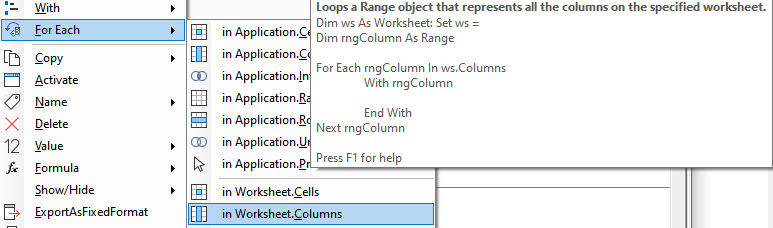﻿ Excel Range Select Columns VBA

# Using the Excel Range Columns property in VBA

The following sections explain how you can use worksheet or range object `.Columns` property. It begins with explaining two ways to select a column. Next, it is explained how to loop over the columns collection.

## Select a column

The `.Columns` property returns a collection of columns. Which columns are to be included is determined by the RowIndex and ColumnIndex properties as explained below. There are three classes that support the Columns property:

• `Application.Columns`: columns on the active worksheet. This is what is assumed when Columns is called without specifying the range or worksheet.
• `Worksheet.Columns`: columns on the specified worksheet.
• `Range.Columns`: columns in the specified range.

There are two ways to identify a single column in the Columns collection:

1. N-th item in the columns collection
2. Select column in the columns collection using column character

Another ways to identify a column is using the range address, e.g. `Range("B:B")`, or `Range("B:D")` for columns B, C and D.

### N-th item in the collection

When leaving out the second optional argument `ColumnIndex`, the Columns property returns the nth item. The order in which items get returned is breadth-first, so in the example table the columns with value a,b,c,...f.

ExpressionValueComment
`Range("B2:C3").Columns(2)`CThe second column in the range
`Range("B2:C3").Columns(3)`DEven though the range only has two columns, ...
Note
Negative values in the index are not allowed.

#### Counting columns and cells

`Range("B2:C3").Columns.Count` returns 2 columns, `Range("B2:C3").Columns.Cells.Count` returns 4 cells.

#### Column in range and EntireColumn

As shown in above example, `Columns` applied to a range only includes the cells in that range. To get the complete column, apply `EntireColumn`.

``Dim rng As Range: Set rng = Application.Range("B2").EntireColumn``

### Select one or more columns in the columns collection using column character

ExpressionColumnComment
`Range("B2:B3").Columns("B")`CColumn character interpreted as number, e.g. "B" is always 2nd column, even if not in the original range
`Range("B2:B3").Columns("B:C")`C and DColumn character interpreted as number, e.g. "B" is always 2nd column

## Loop over columns in Range

The `Columns` property is particularly useful because it makes it easy to iterate over a range. The image below shows the part of the Code VBA (download) menu that lets you insert the code fragment you require.### Loop over all columns in the range

The code below shows how you can loop over all columns in the range. Here it would print values a,b,c,d from the table at the top of the page.

``````Dim rng As Range: Set rng = Application.Range("B2:C3")
Dim col As Range
For Each col In rng.Columns
Debug.Print col.Value
Next col
``````